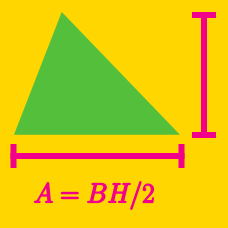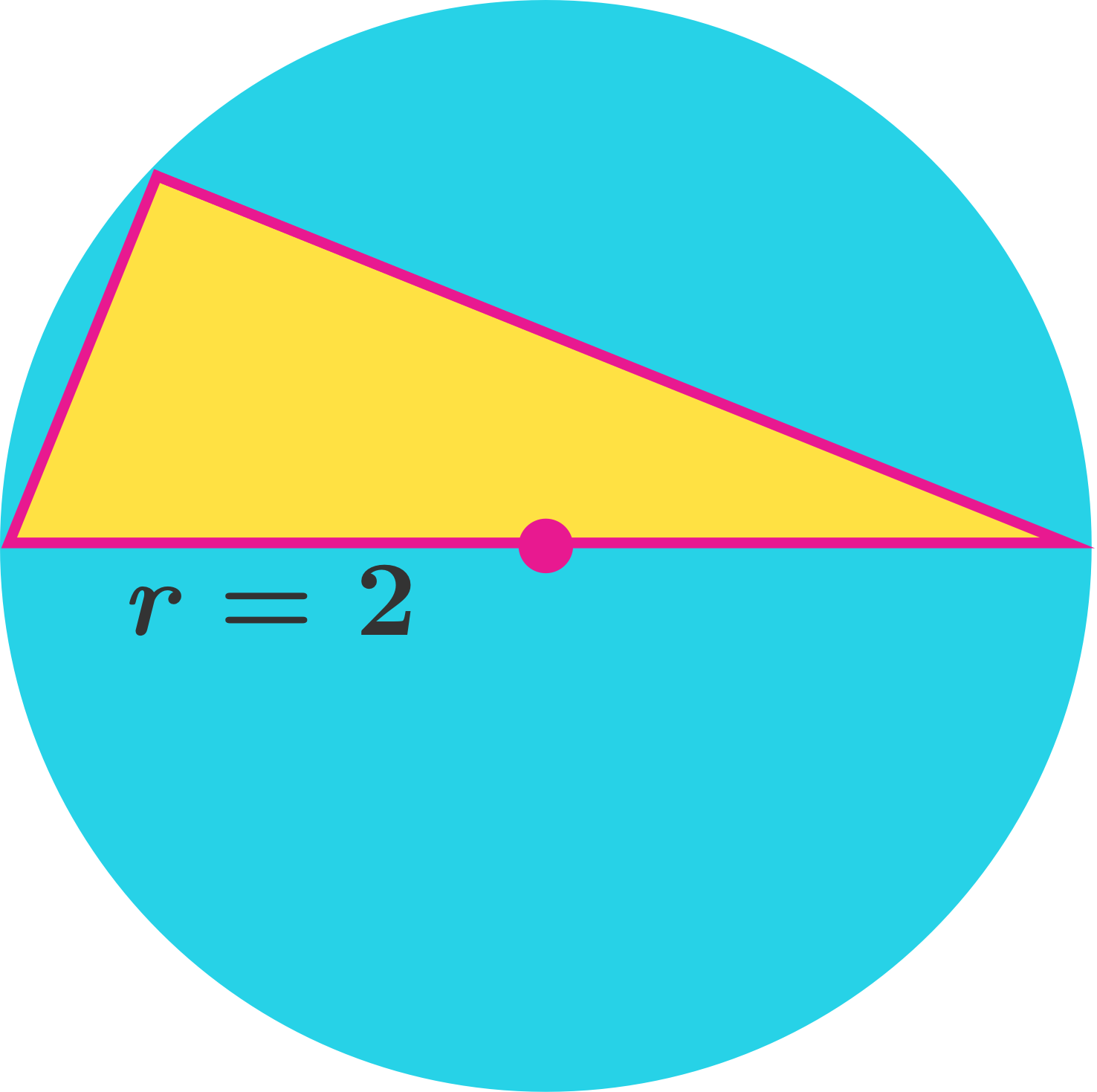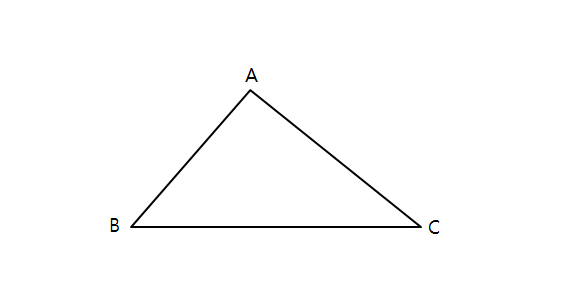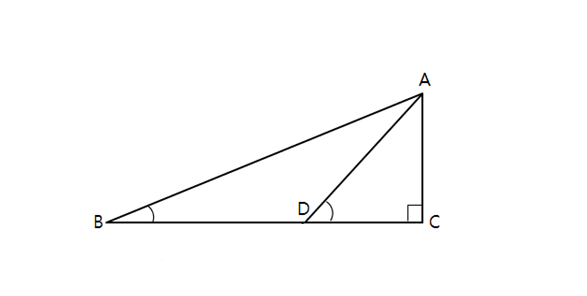Geometry

# Area of Triangles - Problem Solving

If the lengths of the three sides of triangle $\triangle ABC$ sum to $13,$ and the inscribed circle has radius $8,$ what is the area of triangle $\triangle ABC?$

Consider a triangle inscribed in a circle with radius $2.$ If one side of the triangle is a diameter of the circle, what is the largest possible area of the triangle?In the above diagram, we are given two side lengths $\rvert \overline{AB} \lvert = 3, \rvert \overline{AC} \lvert = 9.$ If $\sin(\angle B+ \angle C) = \frac{1}{4},$ what is the area of $\triangle ABC?$

Note: The above diagram is not drawn to scale.In the above right triangle, if $\angle ABC = 30^{\circ}, \angle ADC = 45^{\circ}, \lvert \overline{BC} \rvert = 14,$ what is the area of $\triangle ADC?$

Triangle $ABC$ has vertices $A=(-4,k),$ $B=(5,0)$ and $C=(4,6).$ If the area of triangle $ABC$ is $23$, what are the possible values of $k$?

×